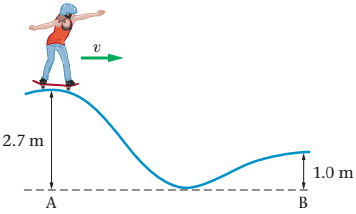# Find final speed using mechanical energy formula

• Angela_vaal

## Homework Statement

A skateboarder at a skate park rides along the path shown in (Figure 1) .If the speed of the skateboarder at point A is v=1.3m/s, what is her speed at point B? Assume that friction is negligible.ui+ki=uf+kf
u=mgh
k=1/2mv2

## The Attempt at a Solution

m(9.8x2.7)+1/2m(1.3)2=m(9.8x1)+1/2mvf2
m(26.46+.845)=m(9.8+.5) mvf2
vf=√((26.46+.845)/(9.8+.5))
The answer is wrong. Can somebody please tell me what I am doing wrong? Thanks!

Last edited by a moderator:

Looks like you changed 0.845 to 8.45 from one step to the next. That could have caused a problem but I didn't verify that was it.

Looks like you changed 0.845 to 8.45 from one step to the next. That could have caused a problem but I didn't verify that was it.

I wrote it down wrong here but I did the work using.845 and still got 1.6m/s. I don't know what I am doing wrong :(

I'll look at it again.

Yeah, you have a math problem in going from the first line to the second line. You should re-work that again carefully.

Yeah, you have a math problem in going from the first line to the second line. You should re-work that again carefully.
I will do that right now.

I"m not that great at math. Can you give me a hint of what I did wrong?

@Angela_vaal you wrote:
m(9.8x2.7)+1/2m(1.3)2=m(9.8x1)+1/2mvf2
It's okay that you didn't, but at this point you could have (probably should have) canceled out all of the m's, because each term contains an 'm'.

Then you wrote:
m(26.46+.845)=m(9.8+.5) mvf2
I'm not exactly sure what you did to get to this equation. It looks like you're trying to factor mvf2 out of both terms on the right-hand side of the equation, but the first term does not contain a vf2. So that was not legal.

I think you ought to factor out all of the 'm's in the first equation, multiply out the numbers, and that will [hopefully] make it clearer to see how to proceed.

ui+ki=uf+kf
u=mgh
k=1/2mv2

## The Attempt at a Solution

m(9.8x2.7)+1/2m(1.3)2=m(9.8x1)+1/2mvf2
m(26.46+.845)=m(9.8+.5) mvf2
The RHS should be 9.8m+0.5 mvf2
Factor out m.

Last edited:
Yeah I think it is just a math error as the general method of solving looks fine

I figured it out thank you!